## Content

### Behaviour of polynomials at infinity

Understanding the behaviour of a polynomial function $$f(x)$$ when $$x$$ is large (that is, as $$x \to \pm \infty$$) helps us to sketch the graph of $$y=f(x)$$.

We've seen from examples that, for polynomial functions up to degree 4, the graph of a polynomial $$f(x) = a_n x^n + a_{n-1} x^{n-1} + \dots + a_0$$ behaves asymptotically like its leading term $$a_n x^n$$.

Let's consider how this behaviour arises. Take a cubic polynomial that we saw earlier, $$f(x) = 2x^3 - 3x^2 + 5$$.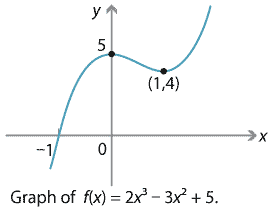Detailed description of diagram

When $$x$$ is large (positive or negative), $$x^2$$ is much larger again, and $$x^3$$ dwarfs them both. So the $$x^3$$ term will 'dominate' the others. One way to see this algebraically is to write

$f(x) = 2x^3 - 3x^2 + 5 = 2x^3 \Big( 1 - \frac{3}{2x} + \frac{5}{2x^3} \Big).$

When $$x$$ is large, both $$\dfrac{3}{2x}$$ and $$\dfrac{5}{2x^3}$$ become very small, and so effectively the dominant term is $$2x^3$$. This explains why, as seen on the graph,

$f(x) \to + \infty \ \text{as} \ x \to +\infty, \qquad \text{and} \qquad f(x) \to - \infty \ \text{as} \ x \to -\infty.$

A similar argument applies to any polynomial $$f(x) = a_n x^n + a_{n-1} x^{n-1} + \dots + a_0$$: for large $$x$$, the $$a_n x^n$$ term dominates the others.

Looking at the asymptotic behaviour, we can say that the graph $$y=x^n$$ is roughly U-shaped when $$n$$ is even, in the sense that $$x^n \to +\infty$$ as $$x \to \pm \infty$$. The actual graph may have many turning points, but if we imagine 'zooming out' and looking only at the large-scale picture when $$x$$ is large, the graph is U-shaped. In a similar way, the graph of $$y=x^n$$ is roughly /-shaped when $$n$$ is odd. The behaviour of a polynomial graph $$y=f(x)$$ when $$x$$ is large will depend on whether the degree of $$f(x)$$ is even or odd, and on the sign of the leading coefficient $$a_n$$. We can summarise the outcomes, along with rough shapes of graphs when $$x$$ is large, in a table.

Graph of a polynomial $$f(x)$$ with leading term $$a_nx^n$$
If $$n$$ is and $$a_n$$ is then as $$x \to +\infty$$ and as $$x \to -\infty$$ so shape is roughly
even positive $$f(x) \to +\infty$$ $$f(x) \to +\infty$$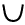even negative $$f(x) \to -\infty$$ $$f(x) \to -\infty$$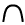odd positive $$f(x) \to +\infty$$ $$f(x) \to -\infty$$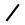odd negative $$f(x) \to -\infty$$ $$f(x) \to +\infty$$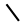This confirms our Conjecture 5.

#### Example

Sketch the graph of $$y= x(x-1)(x-2)(x-3)(x-4)$$, marking all intercepts (but not turning points).

#### Solution

It's clear that the polynomial $$f(x) = x(x-1)(x-2)(x-3)(x-4)$$ has roots at $$x=0,1,2,3,4$$, and so these are the $$x$$-intercepts. The $$y$$-intercept is 0. Also, $$f(x)$$ is a quintic polynomial with leading term $$x^5$$. (This can be seen without fully expanding out the brackets.) Hence $$f(x)$$ behaves like $$x^5$$, for large~$$x$$: as $$x \to + \infty$$, $$f(x) \to +\infty$$, and as $$x \to - \infty$$, $$f(x) \to - \infty$$. This is enough information to sketch the graph.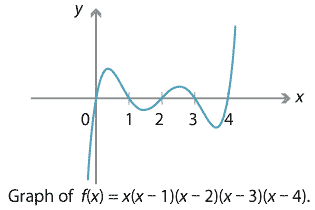Detailed description of diagram

Finally, we can use the asymptotic behaviour of a polynomial $$f(x)$$ to obtain information about its $$x$$-intercepts. When we have an odd-degree polynomial, the graph of $$y=f(x)$$ must go from $$-\infty$$ to $$+\infty$$, or vice versa. Therefore the graph of $$y=f(x)$$ must cross the $$x$$-axis, giving at least one $$x$$-intercept. This proves that the graph of a polynomial of odd degree has at least one $$x$$-intercept, confirming Conjecture 2.

Next page - Content - Stationary points